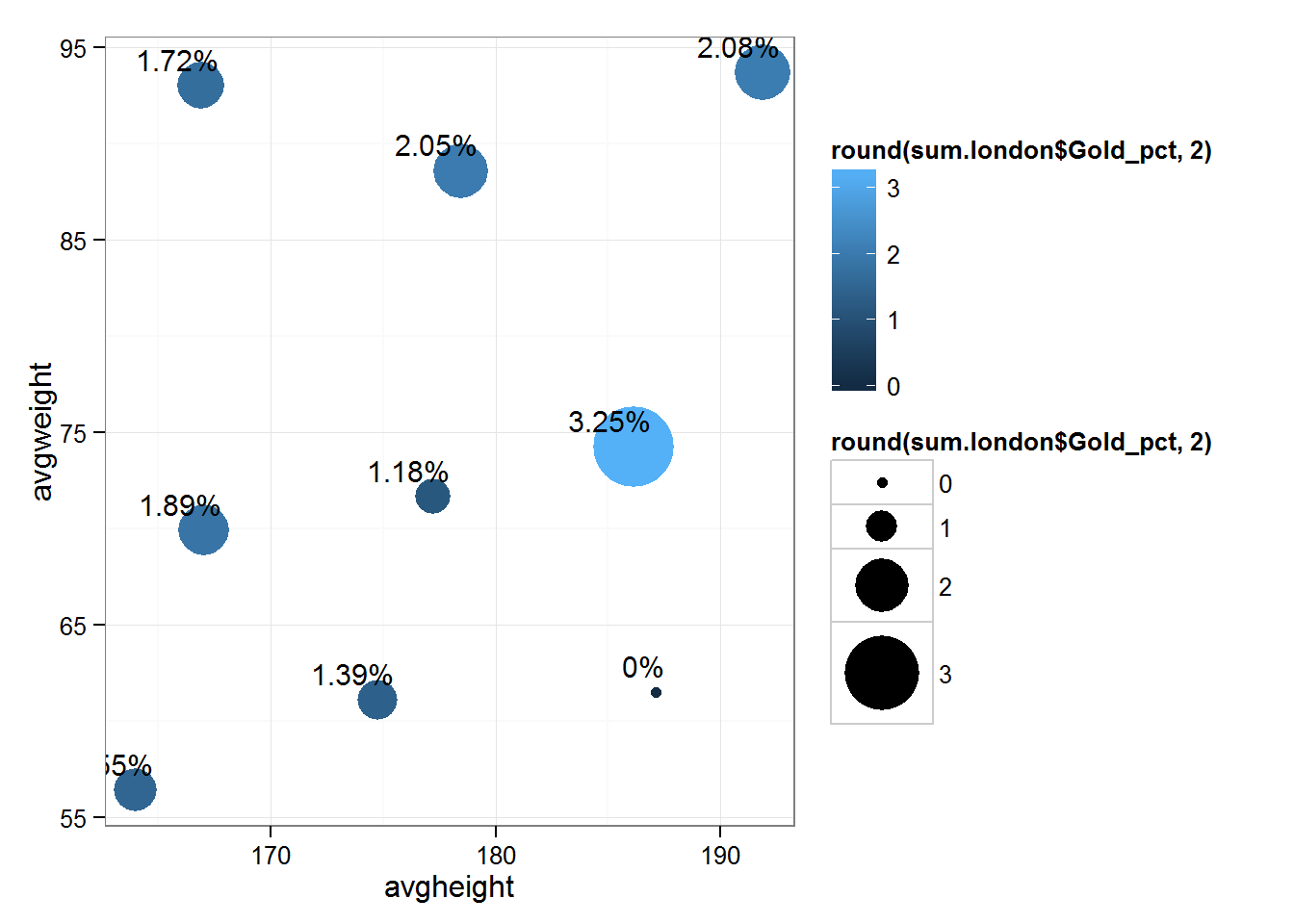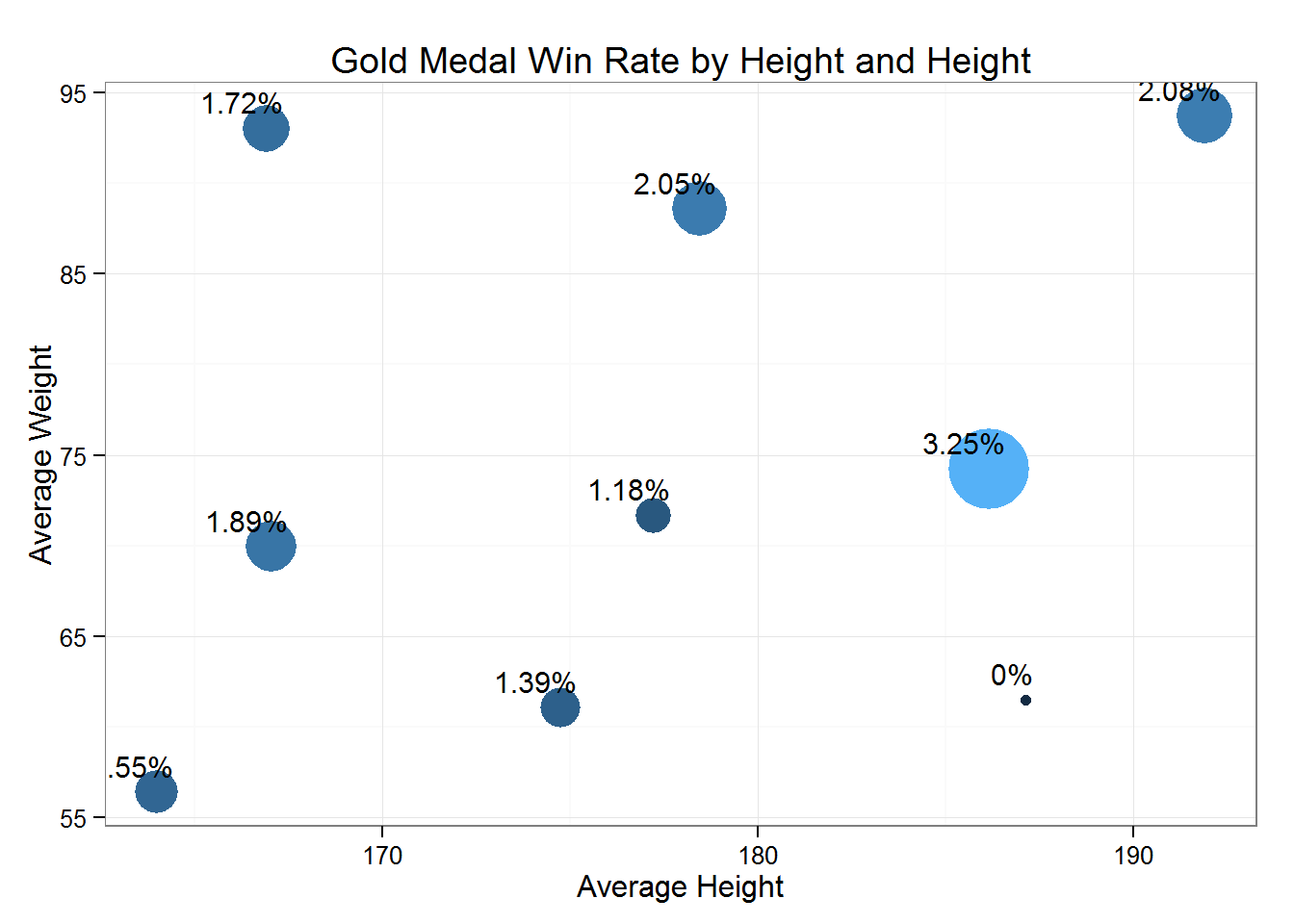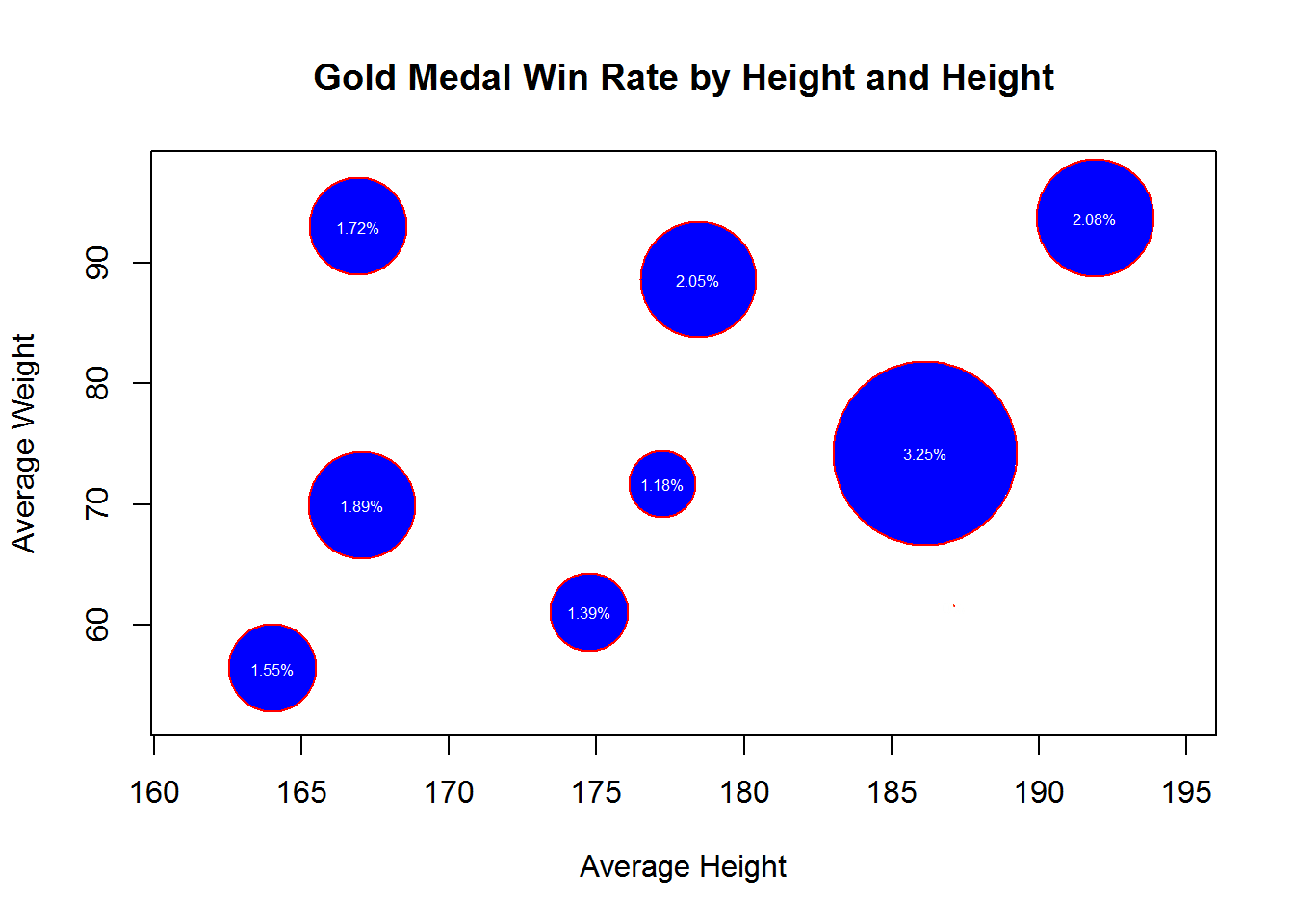# Bubble Chart using ggplot

In this blog, we are going to show plotting of a beautiful bubble chart. A bubble chart is a type of scatter plot. Plotting of Scatter Plot using R

Bubble Chart is used when we want to plot for 3 dimensions. One dimension on X axis, second on Y axis and third is size of bubbles.

In this example, we will use London Olympic Athlete data for Bubble chart plot. We have 3 level of Heights and also Weights. We want to see % winning rate for each of these 9 (3*3) combinations.

Country-wise heatmap is also plotted using R for the athletes.

## Loading required package: tcltk
##   avgheight avgweight Gold_pct
## 1     191.9     93.70    2.083
## 2     187.1     61.51    0.000
## 3     186.2     74.23    3.247
## 4     166.9     93.00    1.724
## 5     164.0     56.40    1.546
## 6     167.1     69.91    1.887
## 7     178.5     88.58    2.052
## 8     174.7     61.07    1.393
## 9     177.2     71.66    1.176

In this blog, we are using ggplot for plottig bubble chart. ggplot can be used for the bubble chart plot.

Note: load ggplot2 library.

geom_point helps in defining bubble size and color geom_text provides coordinates of the label. hjust and vjust values are manipulated to position the label of each bubble. scale_size can be used for defining relative size of the bubble. Wider is the range size difference between low and high value points.

suppressMessages(library(ggplot2))
label <- gsub(" ","",paste(round(sum.london$Gold_pct,2),"%")) ggplot(sum.london, aes(x = avgheight, y = avgweight, label =label ))+ geom_point(aes(size =round(sum.london$Gold_pct,2),color=round(sum.london$Gold_pct,2) ))+ geom_text(hjust = 0.8, vjust=-0.7, size = 4) + scale_size(range = c(2,15)) + theme_bw()##### Axis Labels for a bubble chart As we know ggplot just initialize the ggplot object and all the subsequent steps add layers. Now we want to add labels to the ggplot object, we can use xlab, ylab and ggtitle for X axis, Y Axis and Main Title respectively. Keep in mind that these will be used as a function instead as an option. We also want to remove label of legends. We can use guildes function. label <- gsub(" ","",paste(round(sum.london$Gold_pct,2),"%"))
ggplot(sum.london, aes(x = avgheight, y = avgweight, label =label ))+
geom_point(aes(size =round(sum.london$Gold_pct,2),color=round(sum.london$Gold_pct,2) ))+
geom_text(hjust = 0.8, vjust=-0.7, size = 4) +
scale_size(range = c(2,15)) +
xlab ("Average Height")+
ylab ("Average Weight")+
ggtitle("Gold Medal Win Rate by Height and Height")+
guides(colour=FALSE,size=FALSE)+
theme_bw()Now we are using symbols function for plotting Bubble Chart.

symbols(sum.london$avgheight, sum.london$avgweight,
circles=round(sum.london$Gold_pct,2), inches=0.5, fg="red", bg="blue", xlab="Average Height", ylab="Average Weight", main="Gold Medal Win Rate by Height and Height" ) # Add labels text(sum.london$avgheight,
sum.london\$avgweight,
label,
col="white",
cex=0.5)